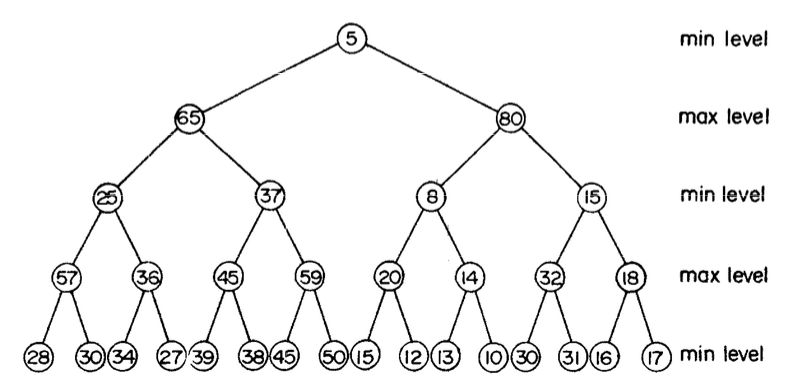Sketchy Polytopes

## Generalized heapsMin-max heaps were introduced in [ASSS86] as an efficient way to support heap operations for both minimum and maximum values. Structurally, the min-max heap levels alternate between min-heap condition and max-heap, and hence evaluates grandchildren/grandparents during insertion or search. Min-max heaps can also be generalized to find the k-th smallest element in $O(1)$ time.

An interesting application is finding the running median of a stream of numbers. [ASSS86] describe a simple extension called min-max-median heap that can find the running median in log-linear time complexity (indexed skip lists can too in amortized time complexity). The following code implements a method for finding the running median on each insertion. The trick is to maintain a min-max and a max-min heap such that either heap has at most one more element than the other.

public double add(int a) {
if (minHeap.size() == 0) {
return a;
}
// add new element to appropriate heap
if (a < minHeap.findMax()) {
} else {
}
int minSize = minHeap.size();
int maxSize = maxHeap.size();
// resize heaps to enforce size constraint
if (maxSize == minSize - 2) {
} else if (minSize == maxSize - 2) {
}
minSize = minHeap.size();
maxSize = maxHeap.size();
// calculate median
if (minSize > maxSize) {
return minHeap.findMax();
} else if (maxSize > minSize) {
return maxHeap.findMin();
}
return (minHeap.findMax() + maxHeap.findMin()) / 2.0;
}


ASSS86 M. D. Atkinson, J.-R. Sack, N. Santoro, and T. Strothotte. Min-max Heaps and Generalized Priority Queues. Communications of the ACM, Vol. 29 No. 10, 1986.